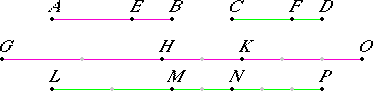# Proposition 17

If magnitudes are proportional taken jointly, then they are also proportional taken separately.
V.Def.14

Let AB, BE, CD, and DF be magnitudes proportional taken jointly, so that AB is to BE as CD is to DF.

V.Def.15

I say that they are also proportional taken separately, that is, AE is to EB as CF is to DF.

Take equimultiples GH, HK, LM, and MN of AE, EB, CF, and FD, and take other, arbitrary, equimultiples, KO and NP of EB and FD.V.1

Then, since GH is the same multiple of AE that HK is of EB, therefore GH is the same multiple of AE that GK is of AB.

But GH is the same multiple of AE that LM is of CF, therefore GK is the same multiple of AB that LM is of CF.

V.1

Again, since LM is the same multiple of CF that MN is of FD, therefore LM is the same multiple of CF that LN is of CD.

But LM was the same multiple of CF that GK is of AB, therefore GK is the same multiple of AB that LN is of CD.

Therefore GK and LN are equimultiples of AB and CD.

V.2

Again, since HK is the same multiple of EB that MN is of FD, and KO is also the same multiple of EB that NP is of FD, therefore the sum HO is also the same multiple of EB that MP is of FD.

And, since AB is to BE as CD is to DF, and of AB and CD equimultiples GK and LN have been taken, and of EB and FD equimultiples HO and MP, therefore, if GK is in excess of HO, and LN is also in excess of MP; if equal, equal; and if less, less.

Let GK be in excess of HO. Subtract HK from each. Therefore GH is also in excess of KO.

But we saw that, if GK was in excess of HO, then LN was also in excess of MP, therefore LN is also in excess of MP, and, if MN is subtracted from each, then LM is also in excess of NP, so that, if GH is in excess of KO, then LM is also in excess of NP.

Similarly we can prove that, if GH equals KO, then LM also equals NP; and if less, less.

V.Def.5

And GH and LM are equimultiples of AE and CF, while KO and NP are other, arbitrary, equimultiples of EB and FD, therefore AE is to EB as CF is to FD.

Therefore, if magnitudes are proportional taken jointly, then they are also proportional taken separately.

Q.E.D.

## Guide

The proposition says that if (w + x) : x = (y + z) : z, then w : x = y : z. Two of the magnitudes w and x can be of one kind while the other two y and z are of another kind.

The converse is given in the next proposition.

This proposition is used in the next two propositions and a couple in Book X.